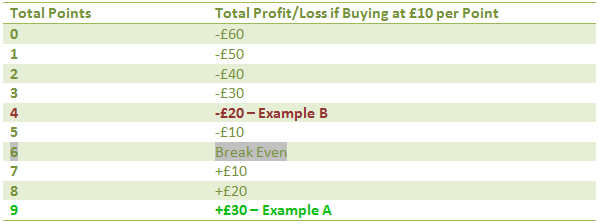# Spread Betting Guide

## Spread Betting Example: Buying 'Total Points'

Our first example below does not use a specific sport, and is intended to help familiarise with the idea of buying and selling against an opening price.

Our chosen opening price is 6 ‘points’. This means in the average game there would be 6 points scored. As an example a stake of £10 is placed on a buy bet:

• Example A: If there were as many as 9 points the bet would return a £30 profit(£10 x 3 points – the difference between the opening price of 6 points and the settlement price of 9 points)
• Example B: If there were only 4 points the bet would lose £20 (£10 x 2 points –the difference between the opening price of 6 points and the settlement price of 4 points).

In example A the buy call is a really good call, with 50% more points than the opening price giving a profit of £30 (from a bet with a total liability of just £60). Example B is not a particularly good call and loses, however does not lose as much as example A wins. This is because it is not as far from the opening price of 6 as example A.

Image 1 shows the full payouts in this example total points marketFor the bet above, as with many spread bets, there is a breakeven point at which there would be no profit or loss. In this case if the settlement price was 6 points then the bet would return zero profit or loss.

To win profit on the buy bet above you would need to pass through the breakeven point and into profit. As 6 points is the breakeven point for every point above this the £10 stake would be paid (as in example A above, £30 would be won if there were 3 points above).

If the break-even point was not reached the loss is £10 stake for each point below (as in example B above, £20 would be lost if there were 2 points below). This means at the point of placing the bet the total liability is £60 (£10 x 6 points). This is the total amount that would be lost if there were no points. Effectively from the start of the game this number will be reduced every time a point is scored, and then paid as profit over 6 points. In example A this happens with a profit of 3 points, however in example B only 4 points are recovered leaving the total loss of 2 points.

Payouts are covered in far more detail, along with selling, in our sections devoted to individual popular football markets. We recommend looking at these after considering liability and total risk on the next page.

Open a new account with Spreadex, stake £300 in qualifying bets and earn an iPad New 32GB.

Francois is one of Howtobet4free’s co-founders and has written many of Howtobet4free’s popular Betting Guides. Francois also helps run the @howtobet4free_ Twitter account